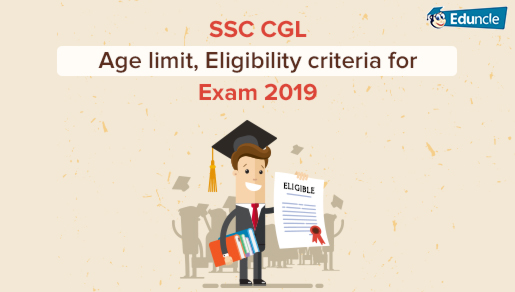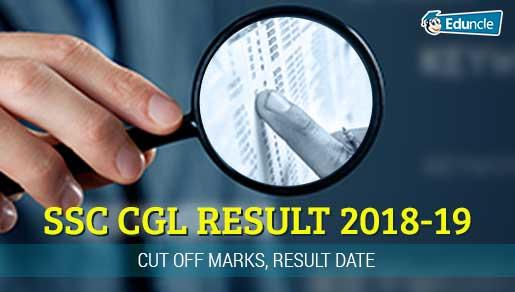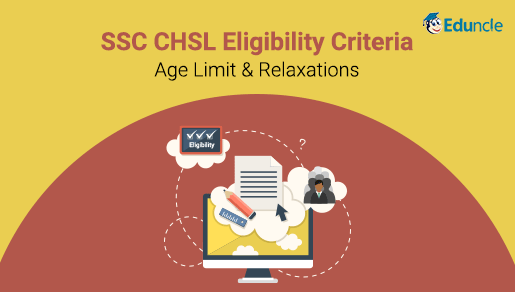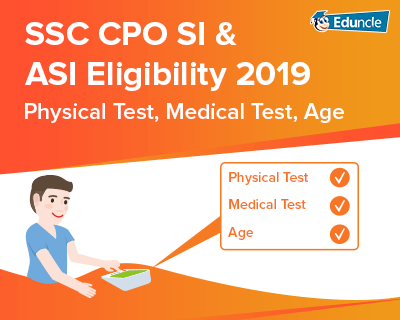SSC FollowShalini Sharma @Eduncle posted an Article
August 21, 2019 • 11:05 am

# Quantitative Aptitude for SSC CGL, CHSL, CPO & GD - Preparation TipsQuantitative Aptitude is one of the indispensable parts of competitive exams. It is one of the most important and scoring sections in various SSC Exams like SSC CGL, SSC CHSL, SSC CPO, SSC GD etc. The weightage of Quantitative Aptitude for SSC CGL Exam is even more as it is the part of both the Tier 1 and Tier 2.

Quantitative Aptitude for SSC Exams can prove to be the most important factor in your selection as an SSC Officer. So, naturally, it requires more preparation time and practice as compared to the other ones.

Download customized study material for SSC CHSL 2018-19 and invest your efforts in the right direction with Eduncle.

## Why is Scoring Well in Quantitative Aptitude for SSC Necessary?

If you score well in the Quantitative Aptitude for SSC Exams, there are high chances that your overall score will be good, and you may get high profile post.

To score well, one should have a good command over the basic concepts and also able to make fast calculations using the short tricks and formulas.

It's a fact that Practice is the key to score well in this section. You must get complete clarity on the fundamental mathematical concepts and then practice with the help of solving questions based on that.

In this blog, we will provide you the broad perspective on how to approach the Quant Section for the SSC Exams.

## Pattern of Quantitative Aptitude Section for SSC Exams

For a better preparation, it is very important to get the details of exam pattern first. Below you can check the pattern, difficulty level and expected number of questions in every SSC Quantitative Aptitude section.

### Quantitative Aptitude for SSC CGL Exam

In Tier 1 There will be 25 questions from the SSC CGL Quantitative Aptitude section. Topic wise questions for the last two years are given in the table below.

 SSC CGL Quantitative Aptitude Topic No. of Questions (2017) No. of Questions (2018) Arithmetic 7 8-9 Algebra 6 2-3 Trigonometry 3 2-3 Geometry 4 3-5 Number System 1 3-4 Data Interpretation 4 5

In Tier 2 Quantitative Aptitude consists of 100 multiple-choice questions and each question carries two marks. The duration of this exam is 2 hours. In this exam, 0.50 marks are deducted as penalty for each wrong answer.

Get the complete syllabus of Quantitative Aptitude for SSC CGL 2018 here - SSC CGL Quantitative Aptitude Syllabus

### Quantitative Aptitude for SSC CHSL Exam

The weightage of Quant section in SSC CHSL is 50 marks, which consists of 25 questions. There will be negative marking of ¼ marks for each wrong answer.

 Topic No. of Questions Algebra 3 Percentage/Profit, Loss & Discount 3 Mensuration 3 Geometry 2 Trigonometry 3 Ratio/Allegation/Average 1-2 Time and Work 1-2 Data Interpretation 3-4 Simple Interest & Compound Interest 1 Time, Speed and Distance 1-2 Co-ordinate Geometry 1 Number System 2

Get the SSC CHSL syllabus here - SSC CHSL Quantitative Aptitude Syllabus

### Quantitative Aptitude for SSC CPO Exam

SSC SI & ASI written exam includes Paper I (Pre) & Paper II (Mains). Both papers are conducted online.

In Paper I, there are 50 questions of 50 marks in Quantitative Aptitude with negative marking of 0.25 marks for every wrong answer. The topic wise questions for the last year paper is give below:

 Topic No. of Questions Data Interpretation 10 Geometry 10 Trigonometry 5 Algebra 4 Trigonometry 3 Time, Speed & Distance, Work & Time 9 Profit, Loss & Discount 4 Average, Mixture, Allegation 3 SI/CI & Percentage 4 Ratio & Proportion 1

Get the complete syllabus for the SSC CPO here - SSC CPO Syllabus 2018

### Quantitative Aptitude for SSC GD Constable Exam

The Quantitative Aptitude for SSC GD Exam has 25 questions of 25 marks. Matriculation level questions will be asked in the exam.

The topics which can be asked in the SSC GD Exam are:

Averages

Decimals and Fractions

Fundamental arithmetical operations

Percentages, Profit & Loss

Time & Distance; Time & Work

Number Systems

Mensuration

Computation of Whole numbers

Get the complete syllabus for the SSC GD Constable Exam here- SSC GD Syllabus 2018.

Download Sample Study Material and chase Success for SSC CGL 2018-19 with Eduncle.

### Important Topics Asked in SSC ExamsThere are certain topics, whose weightage for the SSC Exams are quite higher as compared to the other ones. To maximize your score, you need to prepare these topics very diligently. Below are some topics from where questions are asked.

### Trigonometry in SSC Exams

This topic is totally based on the formulas. You need to remember as many formulas as you can. Below is the standard table for the values of different angles in TrigonometryImportant Pythagorean Identities

sin2 θ + cos2 θ = 1

tan2 θ + 1 = sec2 θ

cot2 θ + 1 = cosec2 θ

### Geometry for SSC Exams

This section plays quite impactful role in SSC Exams. The level of questions can be easy but tricky. You can focus on a few important topics as

Properties of Lines and Angles:

Straight line: A straight line has neither start nor end and is of infinite length.

Acute Angle: The angle that is between 0° and 90° is an acute angle.

Obtuse Angle: The angle that is between 90° and 180° is an obtuse angle.

Right Angle: The angle that is 90° is a Right angle.

Supplementary angles: If the sum of the two angles is 180° then the angles are called supplementary angles.

Complementary angles: If the sum of the two angles is 90° then the two angles are called complementary angles.

Vertically opposite angles: When two lines intersect, the angles formed opposite to each other at the point of intersection is called vertically opposite angles.

Perpendicular lines: When there is a right angle between two lines, the lines are called perpendicular to each other.

Parallel lines: The line which does not intersect with each other in the entire space is called parallel lines.

Properties of Triangle:

Sum of the all the three angles is 180°.

The sum of any two sides is always greater than the length of the third side.

The difference between any two sides is always less than that of the third side.

An exterior angle is equal to the sum of the interior opposite angles.

The side opposite to the greatest angle is the greatest side and the side opposite to the smallest angle is the shortest side.

Area of a triangle is given by: 1/2XBaseXHeight

Properties of Rectangle:

Each of the interior angles of a rectangle is 90°.

The diagonals of a rectangle bisect each other.

The opposite sides of a rectangle are parallel and equal.

A rectangle whose side lengths are a and b have area= aXb.

A rectangle whose side lengths are a and b have perimeter=2a+2b.

Properties of Circle:

If two chords of a circle are equal, their corresponding arcs have equal measure.

Equal arcs subtend equal angles at the center.

A line from the center and perpendicular to a chord bisects the chord.

Equal chords of a circle are equidistant from the center.

When two circles touch, their centers and their point of contact are collinear.

Two tangents drawn on a circle from the point outside are equal in length.

A tangent to a circle is at a right angle to the radius at the point of contact.

Conquer the art of mastering Quant for SSC CGL & CHSL exams! Practice with Previous Year Papers. Download SSC Question PDFs NOW!

### Data Interpretation

Questions from data interpretation contain figures and graphs. This section is scoring one but consumes lot of time. To solve this section, you have to improve your speed of calculations. DI Questions in SSC Exams can be faced through various forms like

Tabular Charts

Bar Graphs

Pie Charts

Mixed Graphs

Always keep in your Mind that in the circular Graph or Charts 100% is equal to 360 Degree. The questions will be based on the concepts of Percentage, Average, Approx. Value and Ratio.

### Algebra in SSC Exams

Practice questions from algebra and try to solve them in mind rather than on paper. It will increase your thinking power and it will save your time to solve these questions.

This will not only improve your thinking power but also save time in the examination.

Here are some useful formulae for Algebra.### Profit, Loss & Discount in SSC Exams

It is also a very important section from which questions are asked in the exam. Here are some formulas, which can be helpful for solving questions from this section –

The price, at which an article is sold, is called its selling prices, S.P.

The price, at which an article is purchased, is called its cost price, C.P.

Profit/gain= SP-CP

Profit%= (Profit/CP) X100

SP= 100/ (100+gain%)X CP

CP= 100/ (100+gain%)X SP

If the overall cost price exceeds the selling price of the buyer, then he is said to have incurred loss.

Loss= CP- SP

Loss %= (Loss/CP) X CP

SP= (100-loss%)/100X CP

CP= 100/ (100- loss%)X SP

### Percentage in SSC Exams

This section is also time consuming but possess good weightage of marks. You have to keep in mind the following –

If the price of a commodity increases by R%, then the reduction in consumption so as not to increase the expenditure is: [R/(100+R)] X 100%.

If the price of a commodity decreases by R%, then the increase in consumption so as not to decrease the expenditure is: [R/(100-R)] X 100%.

## Books for the Quantitative Exam for SSC Exams

Good books and study material play an important role in the preparation of any exam. In SSC, practice is a must for the Quantitative Aptitude, which is impossible without reliable books.

The important books for SSC Exam can be checked by following the links given below:

 Exams Books SSC CGL SSC CGL Books and Study Material SSC CHSL SSC CHSL Preparation books SSC CPO SSC CPO Preparation Plan & Books SSC GD SSC GD Preparation Tips and Books

## Tips to Score better in Quantitative Aptitude for SSC Exams

There are many aspirants who find it difficult to score well in SSC Exams due to lack of practice and conceptual knowledge. So here we are going to provide you some useful tips to maximize your score:

The rudimentary knowledge creates the base for the higher level of studies. Focus on the basics and formulas of the Quantitative Aptitude.

There are many questions which are repeated every year, so try to solve the previous year papers.

Take every mock test very seriously and solve them in one go within the time constraint.

The knowledge does not help until you practice it. Practice as much as you can, so that solving the questions in the exam becomes easy for you.

Apply short tricks in the mock tests for fast calculations so that you can focus on the other sections.

### Paper Attempting Tips for Quantitative Aptitude for SSC Exams

The SSC Exams are conducted within the limited time constraint, so it is essential to have a strategy to attempt the paper in an effective manner so that you can maximize your score. The following tips will help you to attempt paper in a better way:

Reach the exam centre 1 hour before the commencement of the exam.

Analyze the whole paper first and then attempt the easy questions from it.

After the easy questions, you can go for the typical questions in the ascending order of difficulty.

In the last 5-10 minutes, check the questions in which you have confusion and solve them accordingly.

Don't waste 2 more minutes on any question. If you are unable to solve any question, just leave it.

Focus more on accuracy than the number of attempts. This is equally important along with the number of attempts as there is negative marking in SSC Exams.

Take proper rest before the day of the exam and just revise the formulas.

If you want a job through SSC Exams, you need to prepare Quantitative Aptitude very well. You can take the help from some coaching institutes or go for online studies.

You can share and comment below your queries and views. We will be glad to solve your queries as soon as possible.

All the Best!

Thank You!

You Might Also Like